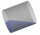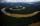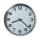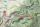# Water flow

How much water flow in pipe with a diameter of 16 cm in 1 hour if the water velocity is 2.5 m/s?

Result

V =  180956 l

#### Solution:Leave us a comment of example and its solution (i.e. if it is still somewhat unclear...):

Showing 0 comments:Be the first to comment!#### To solve this example are needed these knowledge from mathematics:

Do you know the volume and unit volume, and want to convert volume units?

## Next similar examples:

1. Water flow 2How many litres of water will flow in 7 minutes from a cylindrical pipe 1 cm in diameter, if the water flows at a speed of 30 km per hour
2. JarFrom the cylinder shaped jar after tilting spilled water so that the bottom of the jar reaches the water level accurately into half of the base. Height of jar h = 7 cm and a jar diameter D is 12 cm. How to calculate how much water remains in the jar?
3. Water tankThe water tank has a cylindrical shape with a base diameter of 4.2 m and is 80 cm deep. How many minutes will take fill it 10 cm below the edge of the tank if water flowing 2 liters per second?
4. ConvaHow many liters of water fit into the shape of a cylinder with a bottom diameter 20 cm and a height 45 cm?
5. PoolThe pool has the shape of a cylinder with a diameter 4.6m, the presumed height is 130cm. The bucket fits 12l of water. How many buckets do you have to bring to fill this pool?
6. TroughHow many liters of water per second can go via trough, which has a cross section of semicircle with radius 2.5 m and speed of water is 147 cm per second?
7. Cylindrical tank 2If a cylindrical tank with volume is used 12320cm raised to the power of 3 and base 28cm is used to store water. How many liters of water can it hold?
8. AquariumAquarium is rectangular box with square base containing 76 liters of water. Length of base edge is 42 cm. To what height the water level goes?
9. Conserving waterCalculate how many euros are spent annually on unnecessary domestic hot water, which cools during the night in pipeline. Residential house has 129 m of hot water pipelines 5/8" and the hot water has a price of 7 Eur/m3.
10. Circular poolThe 3.6-meter pool has a depth of 90 cm. How many liters of water is in the pool?
11. The shopThe shop has 3 hectoliters of water. How many liter bottles is it?
12. Water wellDrilled well has a depth 20 meters and 0.1 meters radius. How many liters of water can fit into the well?
13. Aircraft nose downHow long will fall airliner from a height of 10000 m at speed 1,000 km/h?
14. WalkersWalker, which makes 120 steps per minute, make distance from point A to point B for 55 minutes. The length of his step is 75cm. For how long does this distance go boy who will do 110 steps 60 cm long in a minute?
15. ClockHow many hours are 15 days?
16. A trip to the peak DryCalculate what percentage is rising trip from Strečno to peak Suchý (Dry peak) longer than the descent back. Team trio of tourists (Palo, Peter and Andrew) rise took 4:21 and descent 3:08.
17. Rotary cylinder 2Base circumference of the rotary cylinder has same length as its height. What is the surface area of cylinder if its volume is 250 dm3?